Next: Beam Parameter Fitting Up: Luminosity Spectrum Previous: Event generation

## Measurement of the Luminosity Spectrum

For Bhabha events, the momentum difference (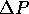) between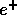- and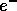-beams can be expressed by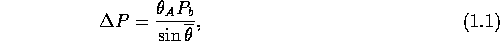where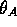is the acollinearity angle,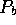is the nominal beam momentum, and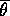is the average ofandpolar angles.

Thus, the momentum difference can be calculated from the acollinearity angles of Bhabha events for the known nominal beam energy. The center-of-mass(CM) energy (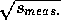) can be calculated using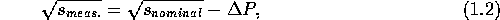where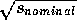is the nominal CM energy, i.e.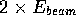for head-on collisions. Sinceis larger than zero,must be less than (or equal to). The beam-energy spread also produces events over the nominal beam-energy, as shown in Figure 14.19(a), while thedistribution is always below the nominal one as shown in Fig14.19(b). This is a limitation of this technique. In order to overcome this limitation for a sizable spread, we need additional information, such as the energies of scattered electrons and positrons.

Now, to separately examine the effects of the finite angular resolution of the detector, the beam energy spread is turned off. The measured spectrum with a perfect detector, i.e. with a perfect angular resolution, is compared with those with angular resolutions (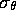) of 3 mrad and 5 mrad in Figure 14.20. In a simulation the polar angles of both tracks are smeared by Gaussian distributions with the assumed angular resolution.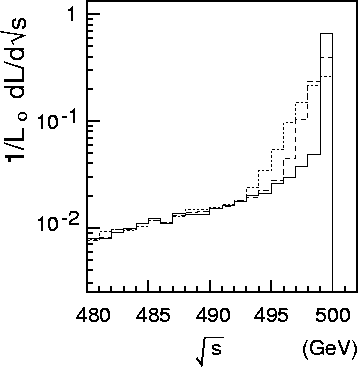Figure 14.20: Luminosity-spectra as measured by the acollinearity angles of the Bhabha events. Various angular resolutions of measuring the Bhabha scattered particles are considered. Solid, dashed and dotted histograms correspond to angular resolutions of=0(perfect), 3mrad and 5mrad, respectively.

The statistics of the simulation in Figure 14.19 corresponds to an accumulated luminosity of 10fb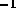(about one year accumulation). The detector is assumed to cover the polar angular region from 45to 135. Since the observed spectrum with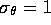mrad resolution is hardly distinguishable from the perfect detector case, it is not independently plotted in Figure 14.20. The spectrum with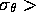3 mrad shows a significant smearing effect.

For studies of toponium physics, accurate knowledge of the luminosity-spectrum within the total decay width of the top quark is very important. The top quark decay width is estimated to be 1.55GeV for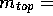175 GeV, i.e.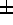0.5% of, according to the standard model. The performance of the accolinearity angle method in this narrow energy region needs to be examined. For this purpose, the integral luminosity for 455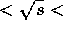450GeV to actually be obtained in collisions, and the luminosity values to be ``measured" by this method are compared. Figure 14.21 shows the results of this study. The ``nominal" (i.e. actual) luminosity is compared with the ``measured" luminosity where various detector angular resolutions and polar angle coverages are assumed. It can be seen that an angular resolution better than 3 mrad is required for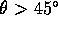to maintain a satisfactory luminosity measurement performance. We also observed a slightly higher luminosity by 2.5% than the nominal one, even with the perfect resolution in Figure 14.21, since a small amount of luminosity must be added in a case that both beams can loose the same energies with no acollinearity angle, where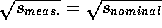, by Equations 14.1 and 14.2.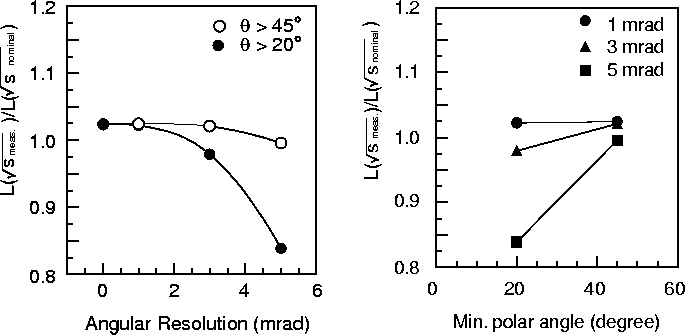Figure 14.22: Luminosity normalized by the nominal one at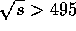GeV as a function of the minimum polar angle to be measured for various angular resolutions.
Figure 14.21: Luminosity normalized by the nominal one atGeV as a function ofin two angular regions of(open circles) and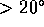(solid circles).

When Bhabha-scattered particles within a smaller polar angle region are included in the analysis the angular resolution of the measurement needs to be substantially improved because of a factor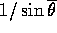in Equation 14.1. On the other hand, the statistics of the Bhabha events increases very rapidly with decreasing the minimum polar angle (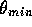) to be measured. For example, the statistics for=20increases by about one order of magnitude compared to that of=45; therefore, the integrated luminosity can be reduced to 1fbin the requirement. As shown in Figures 14.21 and 14.22, the angular resolution must be at least 1 mrad for=20. The toponium experiment will involve an energy scan. It will consist of operations at several energy points each for a few fb. Therefore, it is essential to have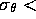1 mrad for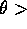20. The higher statistics is also favored in the light of reducing any possible systematic errors in the measurement of the luminosity.Next: Beam Parameter Fitting Up: Luminosity Spectrum Previous: Event generation

Toshiaki Tauchi
Thu, May 29, 1997 04:47:48 PM